4. Seemingly Unrelated Regression
4.1 The Problem
There may be several equations which appear to be unrelated. However, they may be related by the fact that
1. some coefficients are the same
2. the disturbances are correlated across equations
3. a subset of right hand side variables are the same, although all observations are not the same.

The estimator proposed here was developed by Zellner. The classic example uses Grunfeld's investment data. This estimator was the basis for the development of three stage least squares.

4.2 Set Up

We suppose that the ith equation in a set of n equations is given by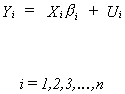where Yi is Tx1, Xi is Txk, bi is kx1, and Ui is Tx1. The whole set of n equations can be written as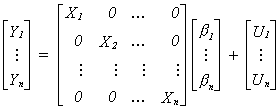or more compactly asThe error variance-covariance matrix is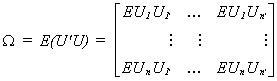(4.1)

Each diagonal term is a TxT variance-covariance matrix for the disturbance of the ith equation. The off-diagonal TxT blocks are contemporaneous and lagged covariances between errors of pairs of equations. We assume the following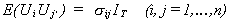(4.2)
For any given equation the disturbance is homoscedastic and non-autocorrelated. For iąj we have non-zero correlation between contemporaneous terms of the two equations, but zero correlation between lagged disturbances. Substituting 4.2 into 4.1 we have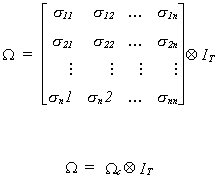Note that W is of full rank.

Caveats

1. If the between equation covariances are zero then there is no advantage to applying Zellner's SUR. It is equivalent to OLS. However, larger covariances mean that there is a larger efficiency gain in using SUR.
2. If the same data is used in each equation, the actual numbers are the same, then there is no advantage to applying Zellner's SUR. It is equivalent to OLS. The more dissimilar the Xs, the greater the gain in using SUR.

Estimation

When W is known we can construct the BLUE estimator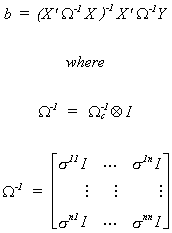The BLU estimator may be written as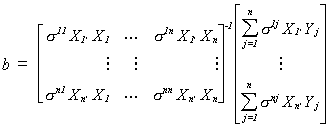As a practical matter we often don't know W and must estimate it. A proposed two step procedure is as follows: 1.a. Fit the n equations separately to obtain the residual vectors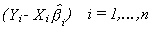1.b. Estimate the variances and covariances as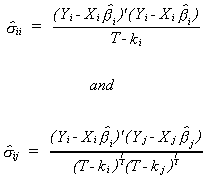1.c. Use the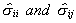to obtain S-1 as an estimate of W-1.

2. Construct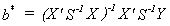The proof from the random effects - error components chapter can be adapted to show when b* is unbiased.

NOTES

1. One could use a likelihood ratio statistic to test for non-zero covariances between equations. The test statistic is
1.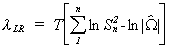where Sn2 is the estimate of the error variance when applying least squares to each equation individually and W is the estimate of the error covariance matrix when there are contemporaneous cross equation covariances. The number of degrees of freedom for this c2 is n(n-1)/2.

2. Occasionally you will see the Zellner estimator iterated. That is, estimate the slopes by OLS, construct an estimate of the error covariance matrix, reestimate the slopes, reestimate the error covariance matrix, and so on. The iterated estimator can be shown to be the maximum likelihood estimator. However, the estimator outlined above is the feasible generalized least squares estimator. Therefore there is no gain from applying the iterations. In large samples the ML and FGLS estimators have the same properties.

An Example

Investment = f1(expected profits)

expected P = f2(value of outstanding stock at end of year)

Ft = market value at end of year

Ct* = desired capital stock where Ct* = bo + b1 Ft

Ct = existing capital stock

By substitution we see that desired net investment is Ct* - Ct = bo + b1 Ft - Ct

Let the fraction of desired net investment that is actually realized be denoted by q1. Then realized net investment is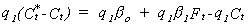Depreciation investment is q2Ct so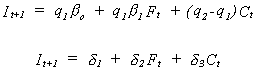We have data on Westinghouse and G.E. for the period 1935-1954. Estimating the parameters for the two companies separately

G.E.: It = -10.0 + .027 Ft-1 + .152 Ct-1 Rbar2 = .671

(.016) (.026)

West: It = -.5 + .053 Ft-1 + .092 Ct-1 Rbar2 = .714

(.016) (.056)

The numbers in parentheses are standard deviations of the coefficients. The estimated error covariances are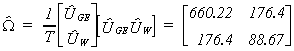Our test statistic for non-zero covariances, with one degree of freedom is

lLR = 20 ( ln(660.22)+ln(88.67) - ln(660.22 x 88.67 - 176.42))

lLR = 15.16

This is a good deal larger than the critical value for any reasonable level of significance so we reject the null hypothesis of diagonal covariance matrix. If we use the fact that the contemporaneous covariances are not zero then the results change slightly to

G.E.: It = -27.7 + .038 Ft-1 + .139 Ct-1

(27.00) (.013) (.023)

West: It = -1.3 + .058 Ft-1 + .064 Ct-1

(7.0) (.013) (.049)

The standard errors of the coefficient estimators have narrowed some. It is also possible to see the efficiency gain in two dimensions.